SNOSD54 June   2017

PRODUCTION DATA.

1. Features
2. Applications
3. Description
4. Revision History
5. Pin Configuration and Functions
6. Specifications
7. Detailed Description
8. Application and Implementation
1. 8.1 Application Information
2. 8.2 Typical Application
1. 8.2.1 Basic Comparator
3. 8.3 System Examples
9. Power Supply Recommendations
10. 10Layout
11. 11Device and Documentation Support
12. 12Mechanical, Packaging, and Orderable Information

• J|14

## 8 Application and Implementation

NOTE

Information in the following applications sections is not part of the TI component specification, and TI does not warrant its accuracy or completeness. TI’s customers are responsible for determining suitability of components for their purposes. Customers should validate and test their design implementation to confirm system functionality.

### 8.1 Application Information

The LM339-MIL device is specified for operation from 2 V to 36 V (±1 V to ±18 V) over the temperature range of 0°C to 70°C. While it may seem like a comparator has a well-defined and somewhat limited functionality as a 1-bit ADC, a comparator is a versatile component which can be used for many functions.

### 8.2 Typical Application

#### 8.2.1 Basic Comparator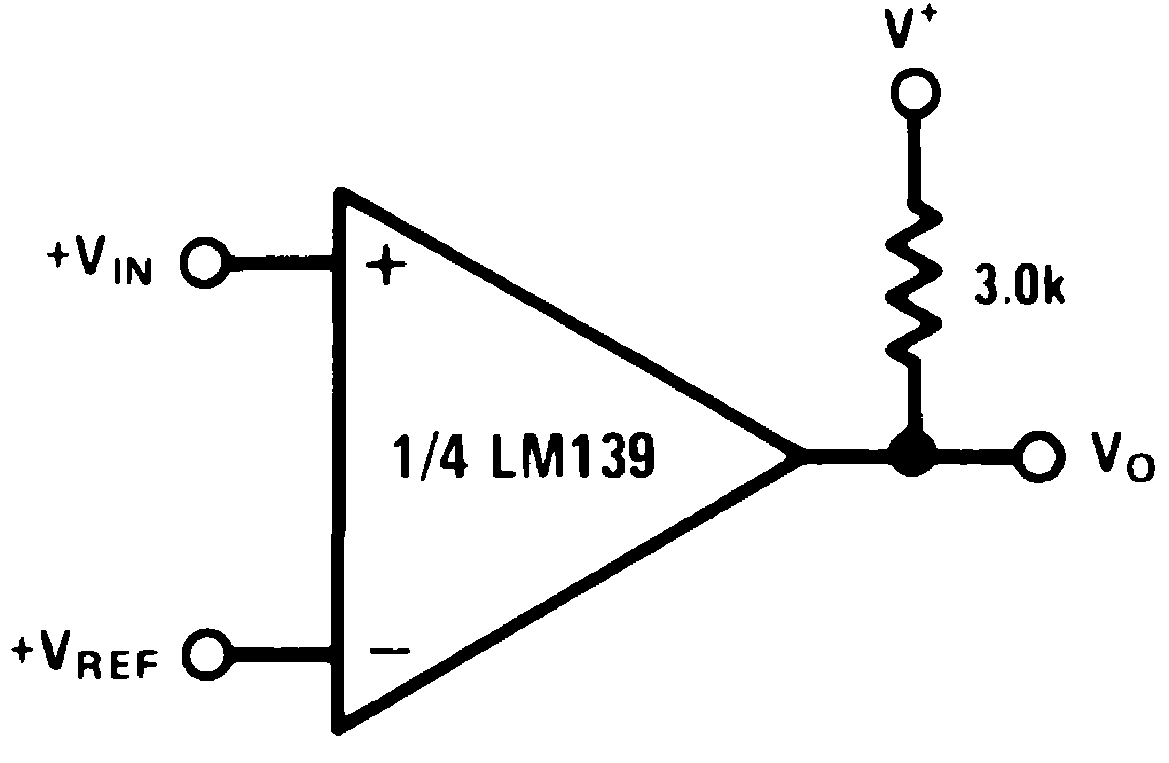Figure 6. Basic Comparator Schematic

#### 8.2.1.1 Design Requirements

The basic usage of a comparator is to indicate when a specific analog signal has exceeded some predefined threshold. In this application, the negative input is tied to a reference voltage, and the positive input is connected to the input signal. The output is pulled up with a resistor to the logic supply voltage, V+.

For an example application, the supply voltage is 5 V. The input signal varies between 1 V and 3 V. Specifically as an example, to know when the input exceeds 2.5 V, set the VREF voltage to 2.5 V.

#### 8.2.1.2 Application Curve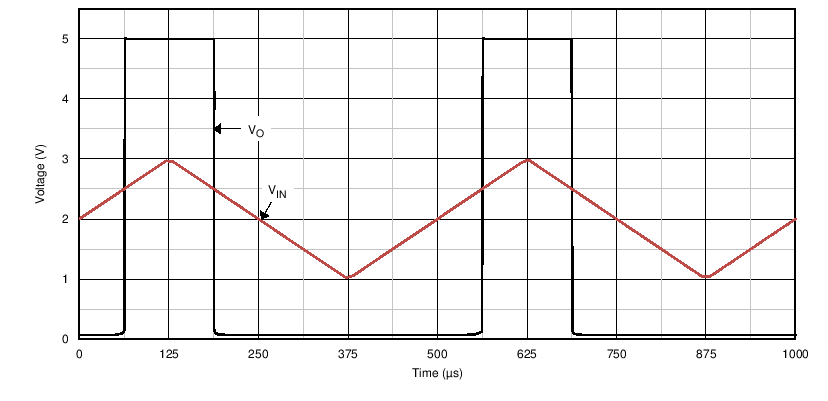Figure 7. Basic Comparator Response

### 8.3 System Examples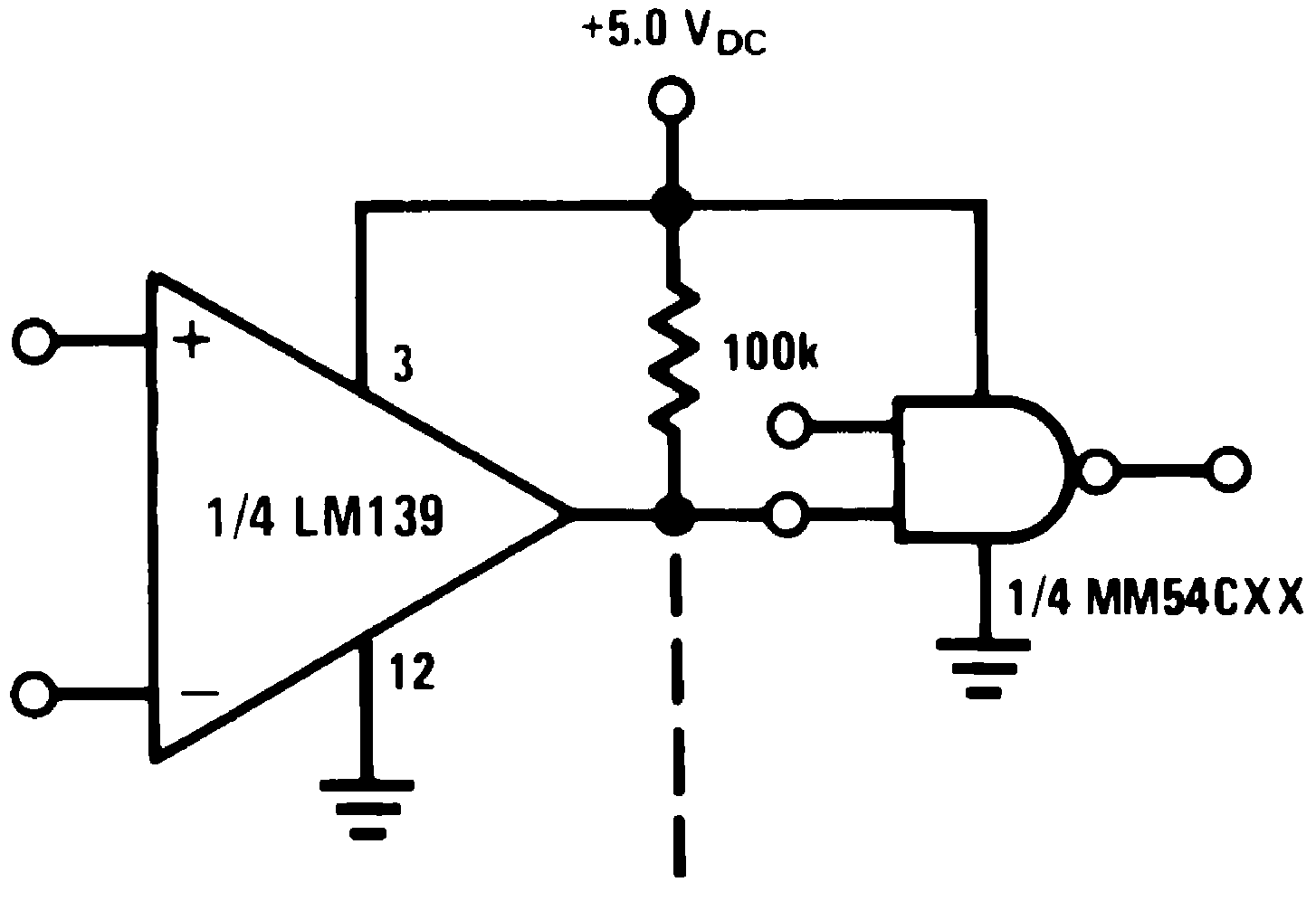Figure 8. Driving CMOS
(V+ = 5 VDC)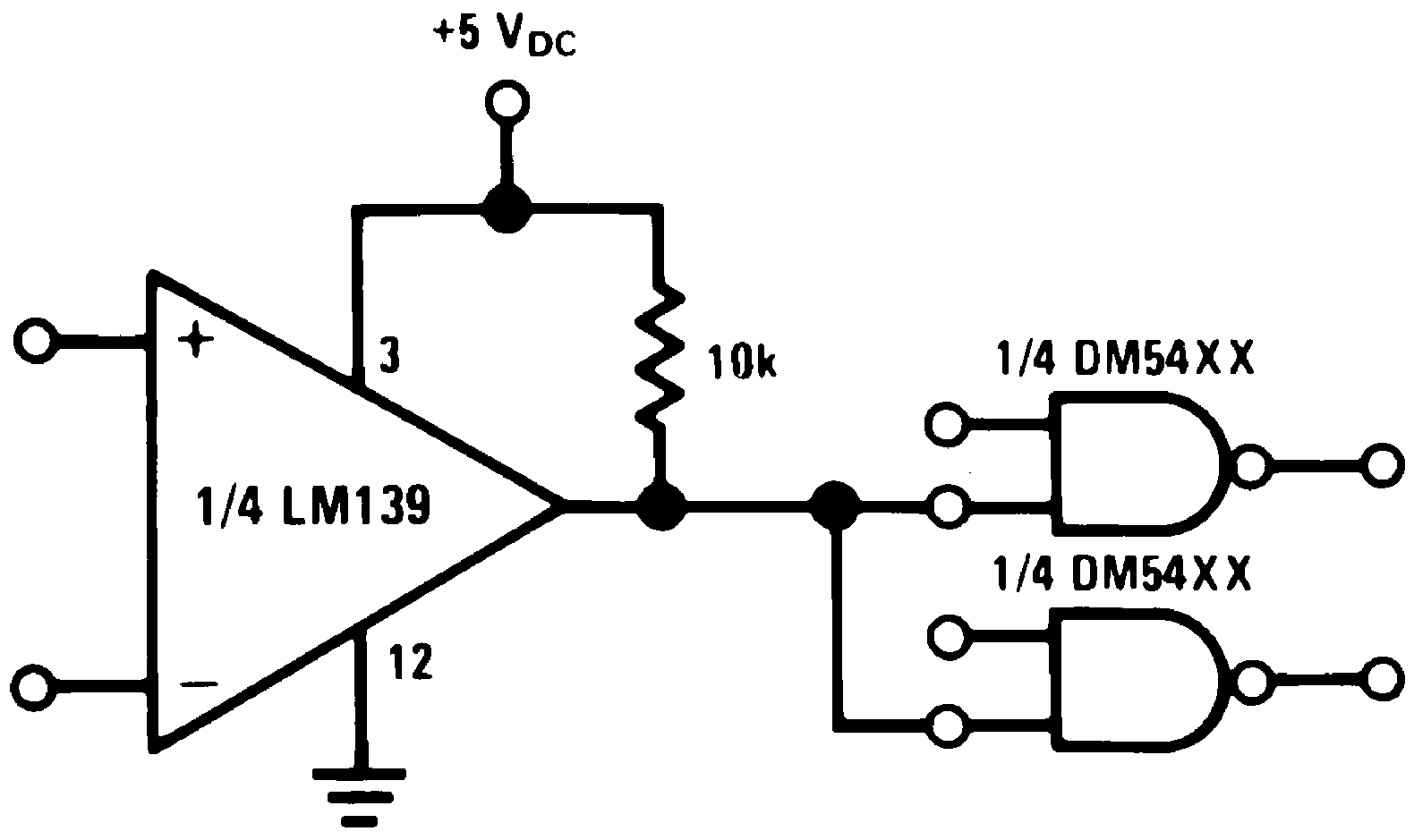Figure 9. Driving TTL
(V+ = 5 VDC)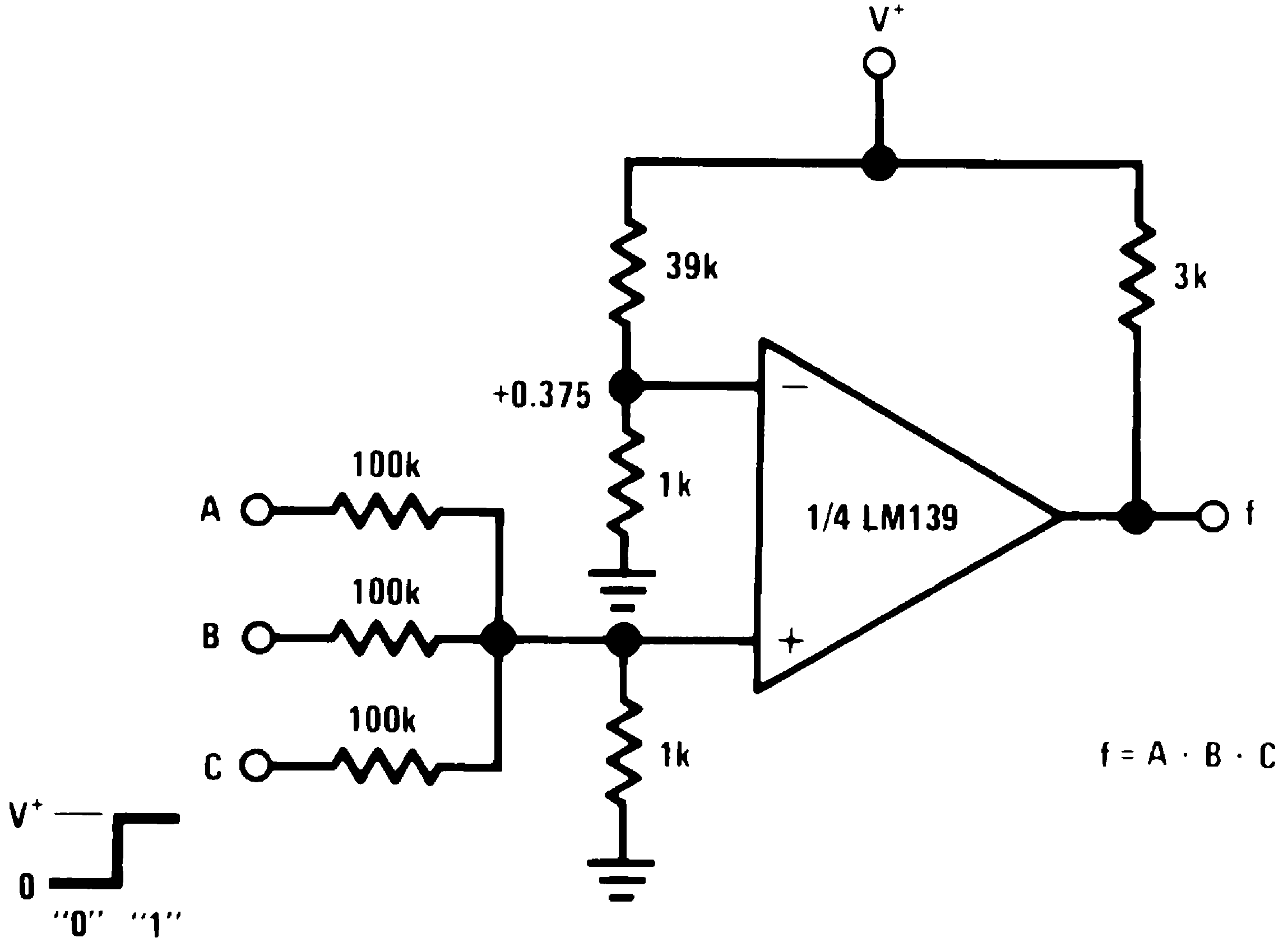Figure 10. AND Gate
(V+ = 5 VDC)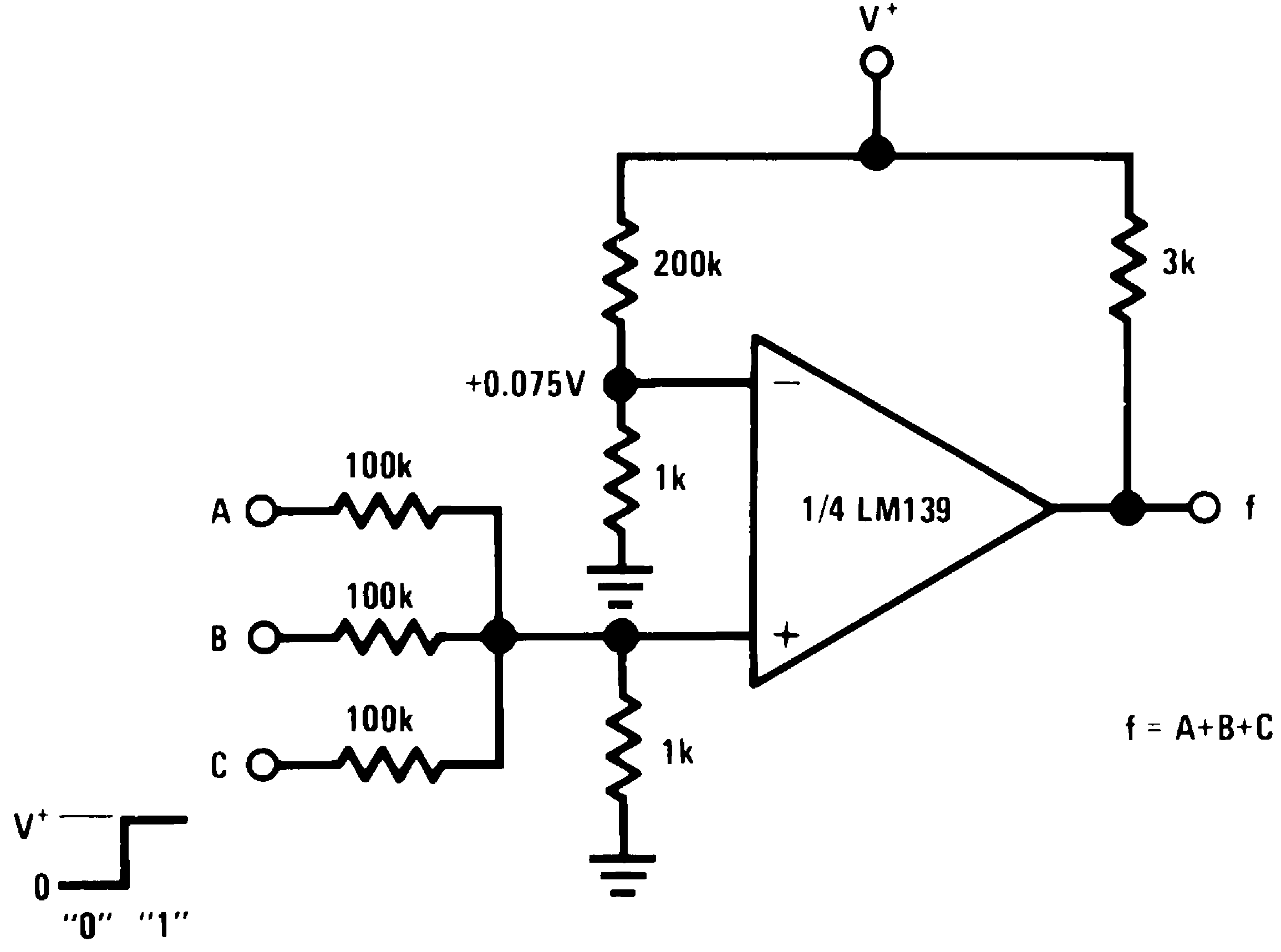Figure 11. OR Gate
(V+ = 5 VDC)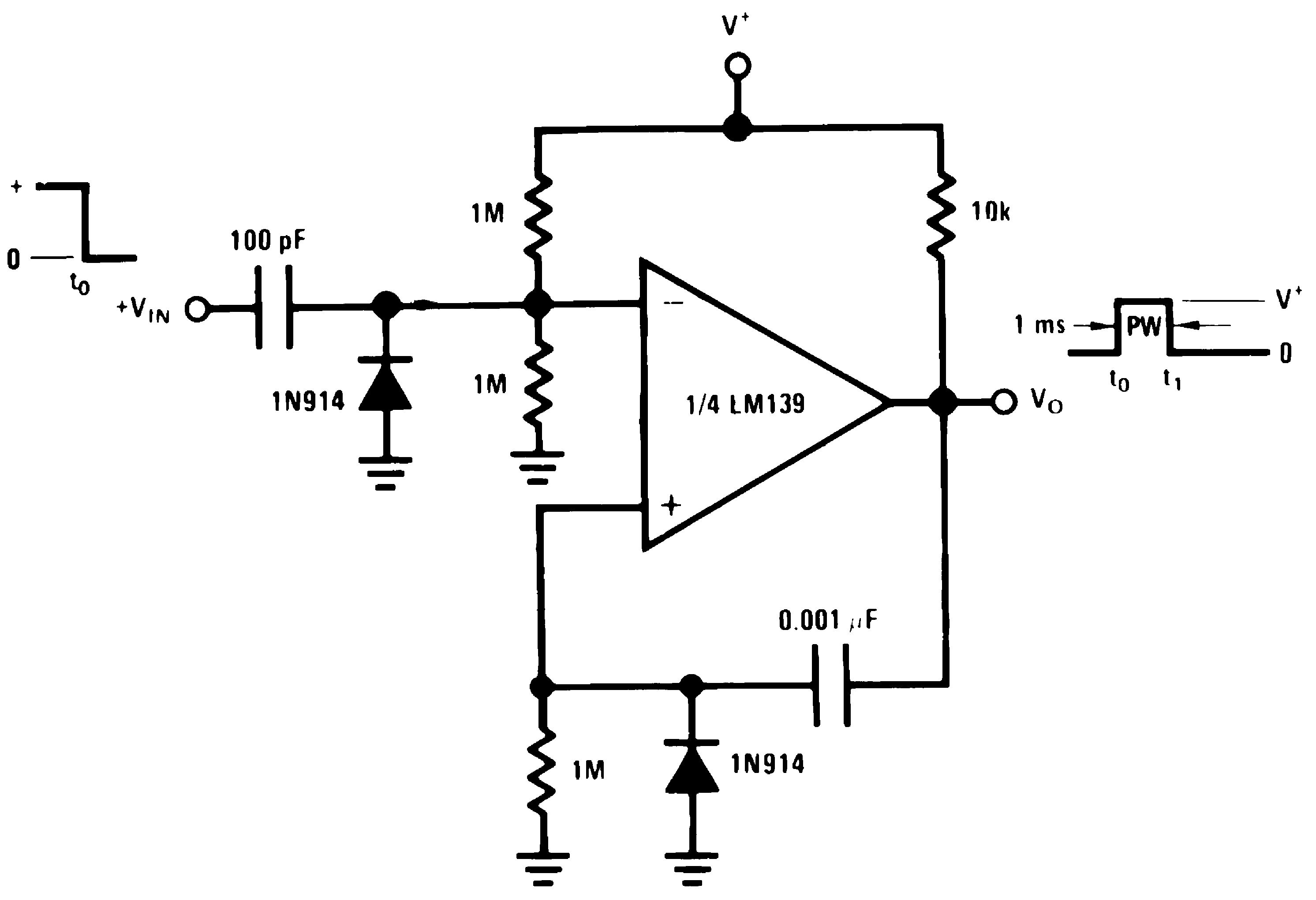Figure 12. One-Shot Multivibrator
(V+= 15 VDC)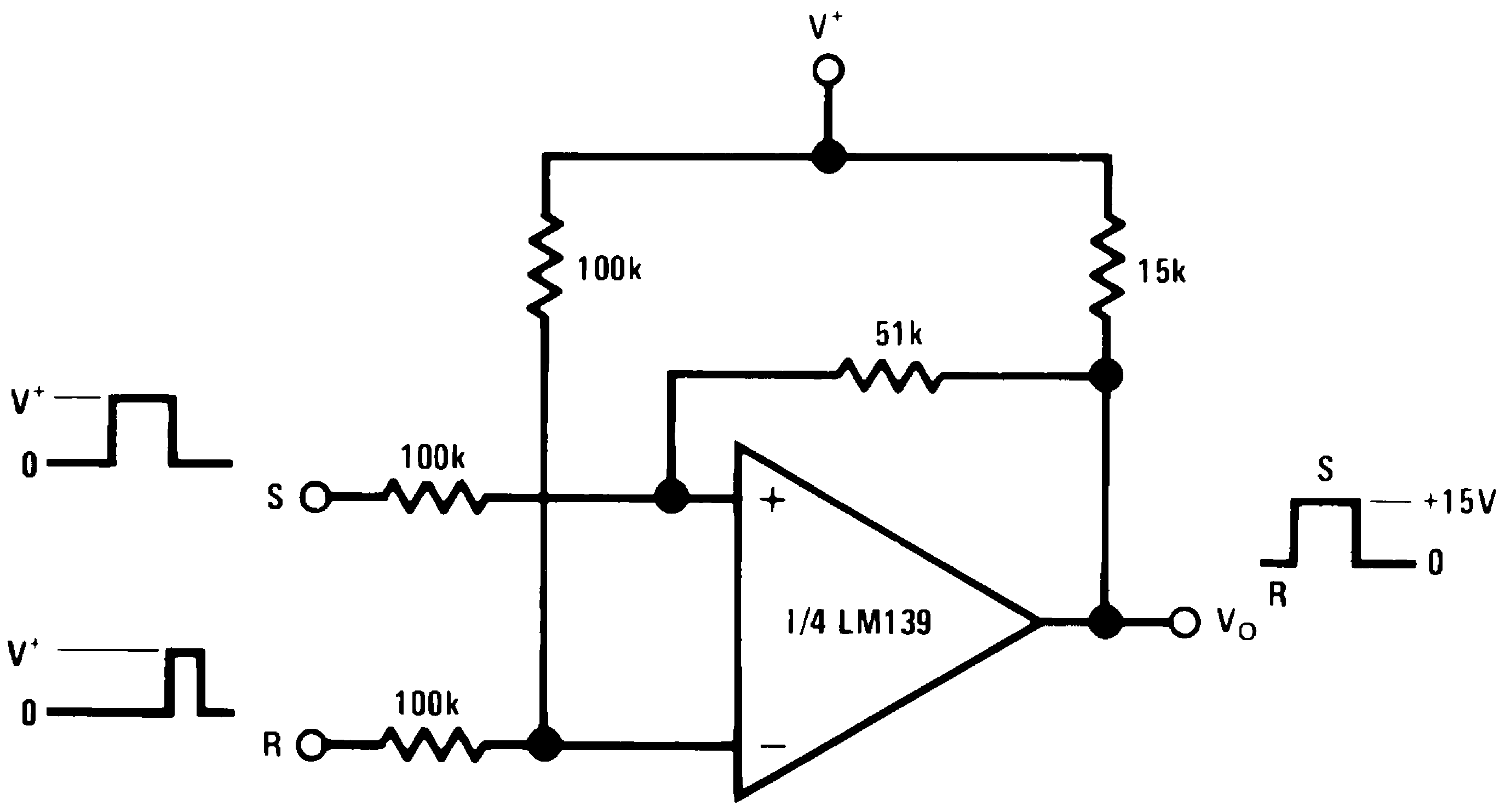Figure 13. Bi-Stable Multivibrator
(V+= 15 VDC)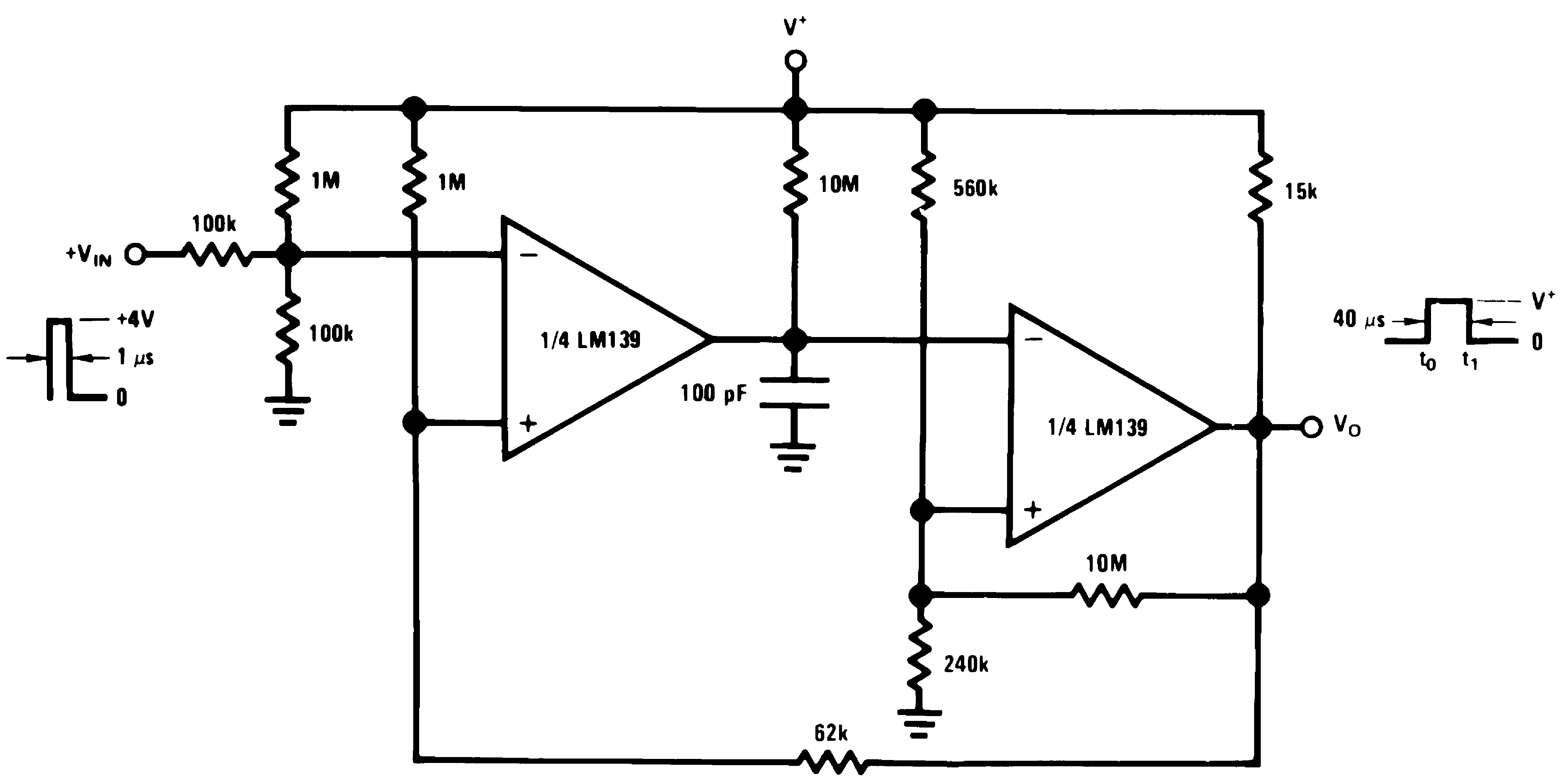Figure 14. One-Shot Multivibrator With Input Lockout
(V+= 15 VDC)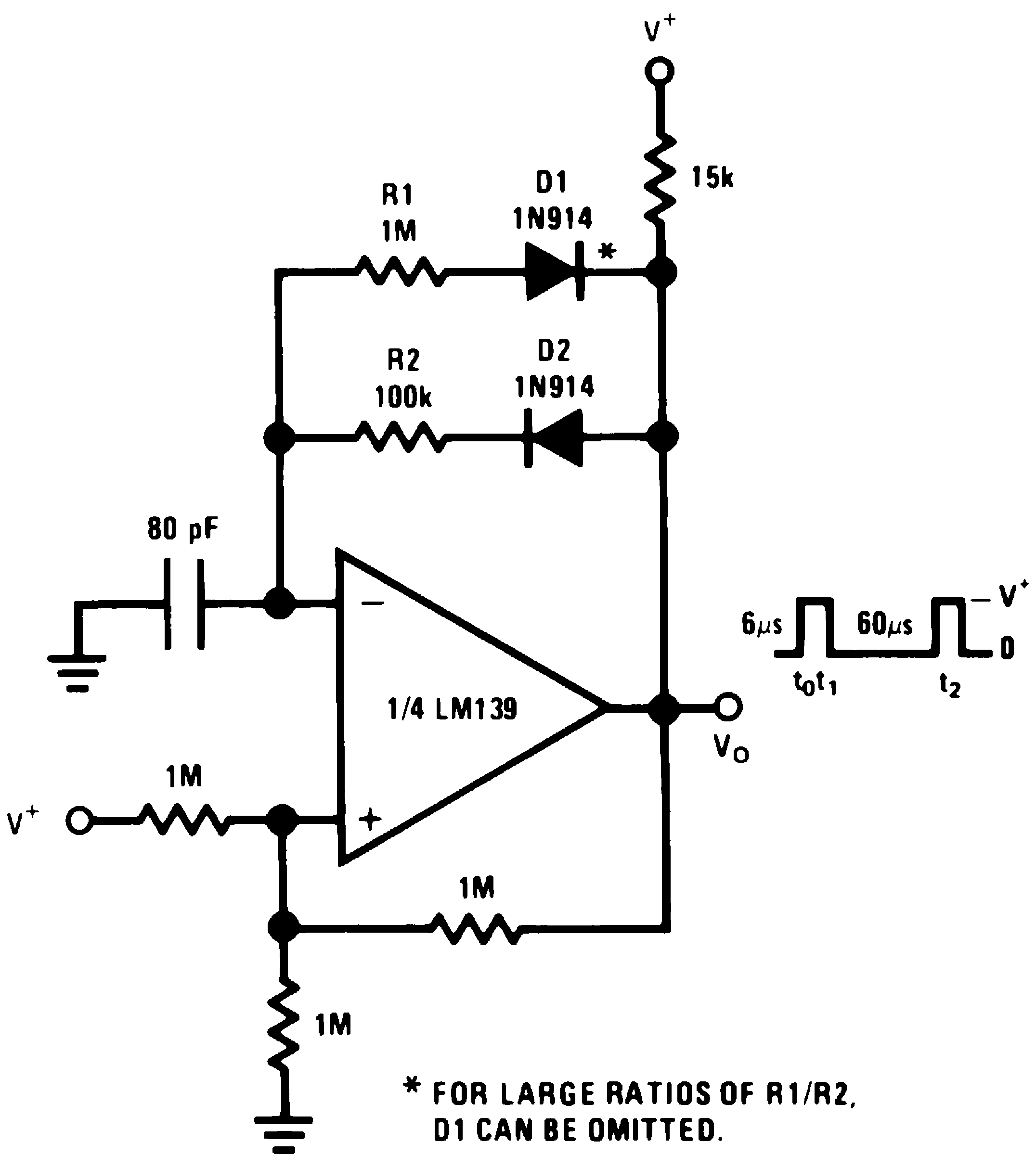Figure 15. Pulse Generator
(V+= 15 VDC)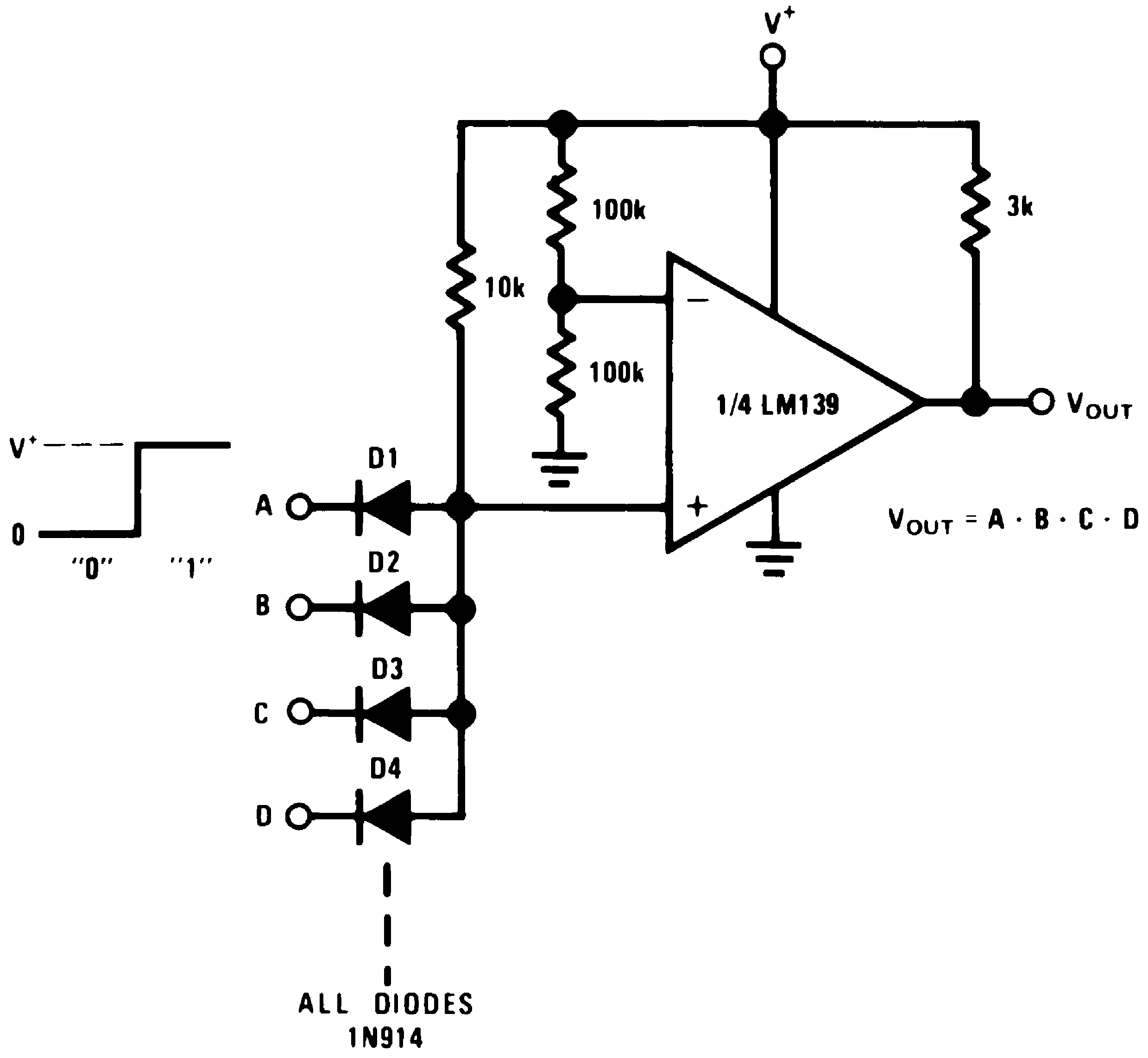Figure 16. Large Fan-In AND Gate
(V+= 15 VDC)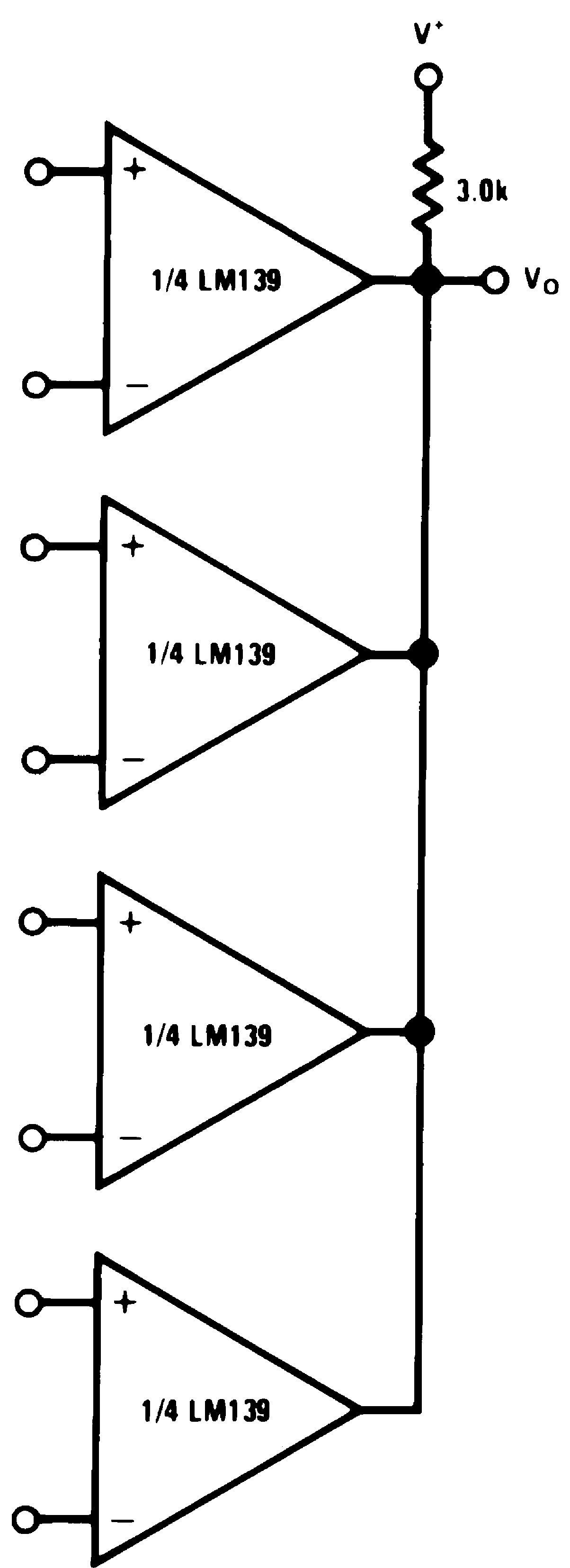Figure 17. ORing the Outputs
(V+= 15 VDC)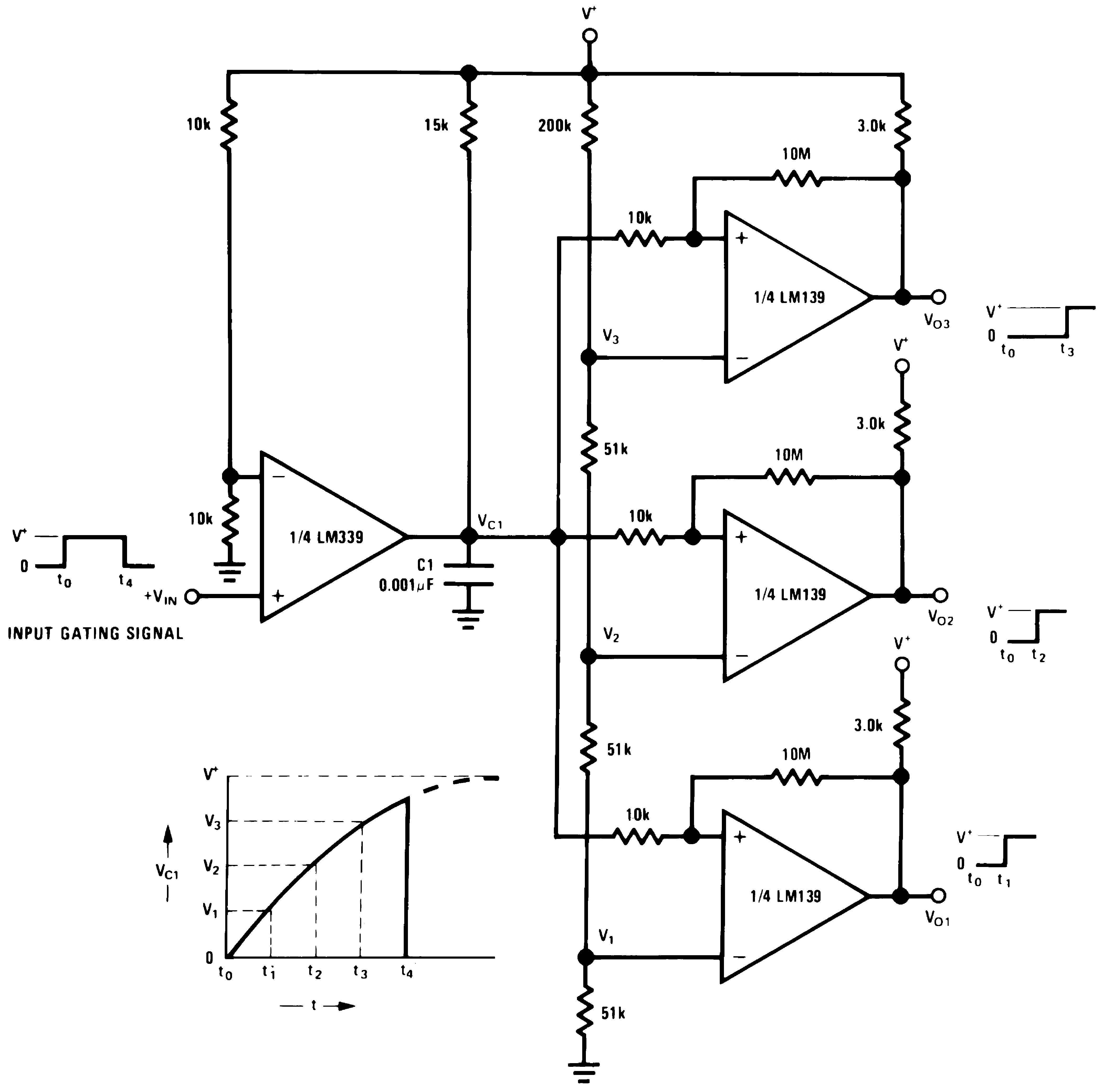Figure 18. Time Delay Generator
(V+= 15 VDC)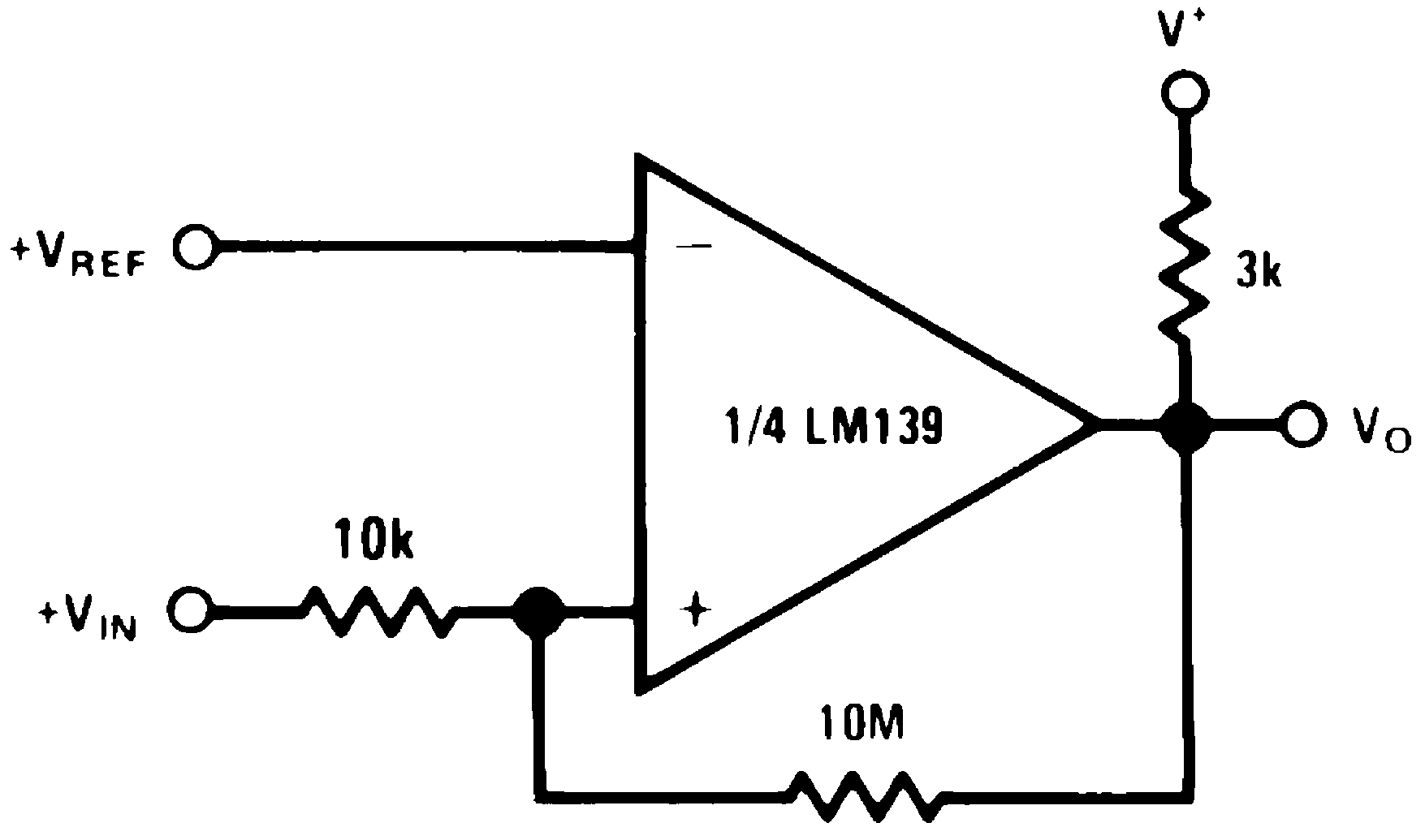Figure 19. Noninverting Comparator with Hysteresis
(V+= 15 VDC)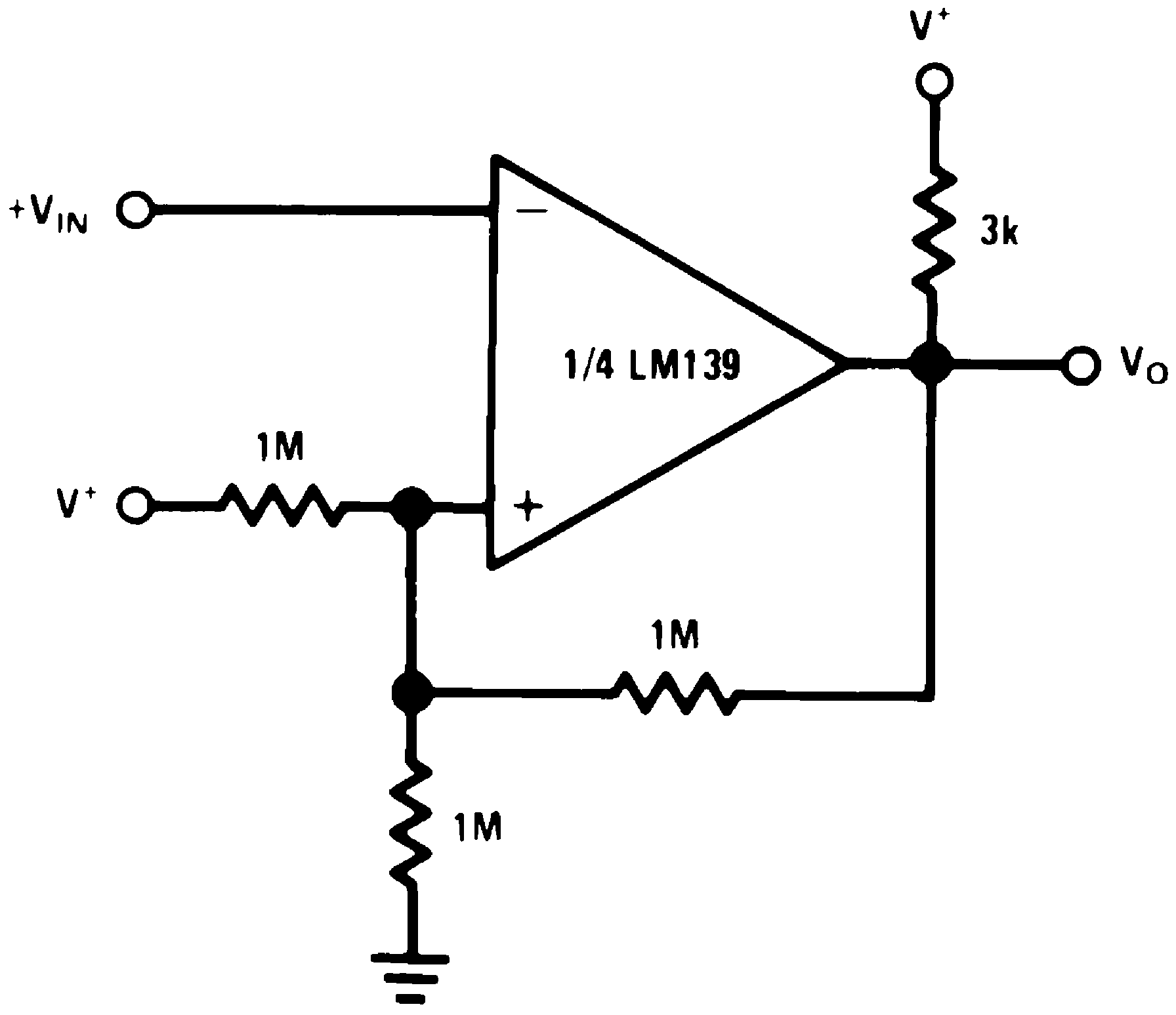Figure 20. Inverting Comparator With Hysteresis
(V+= 15 VDC)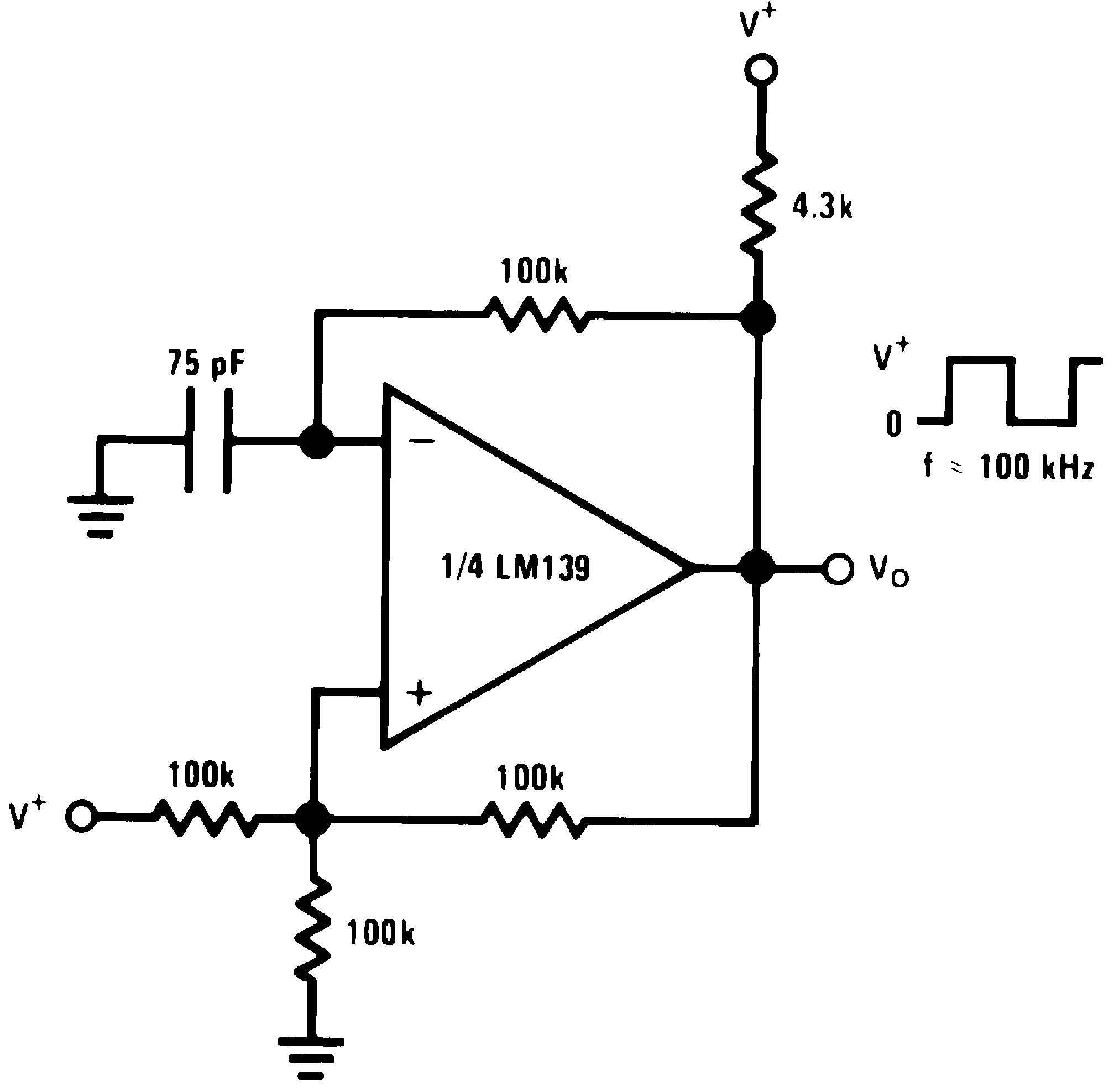Figure 21. Squarewave Oscillator
(V+= 15 VDC)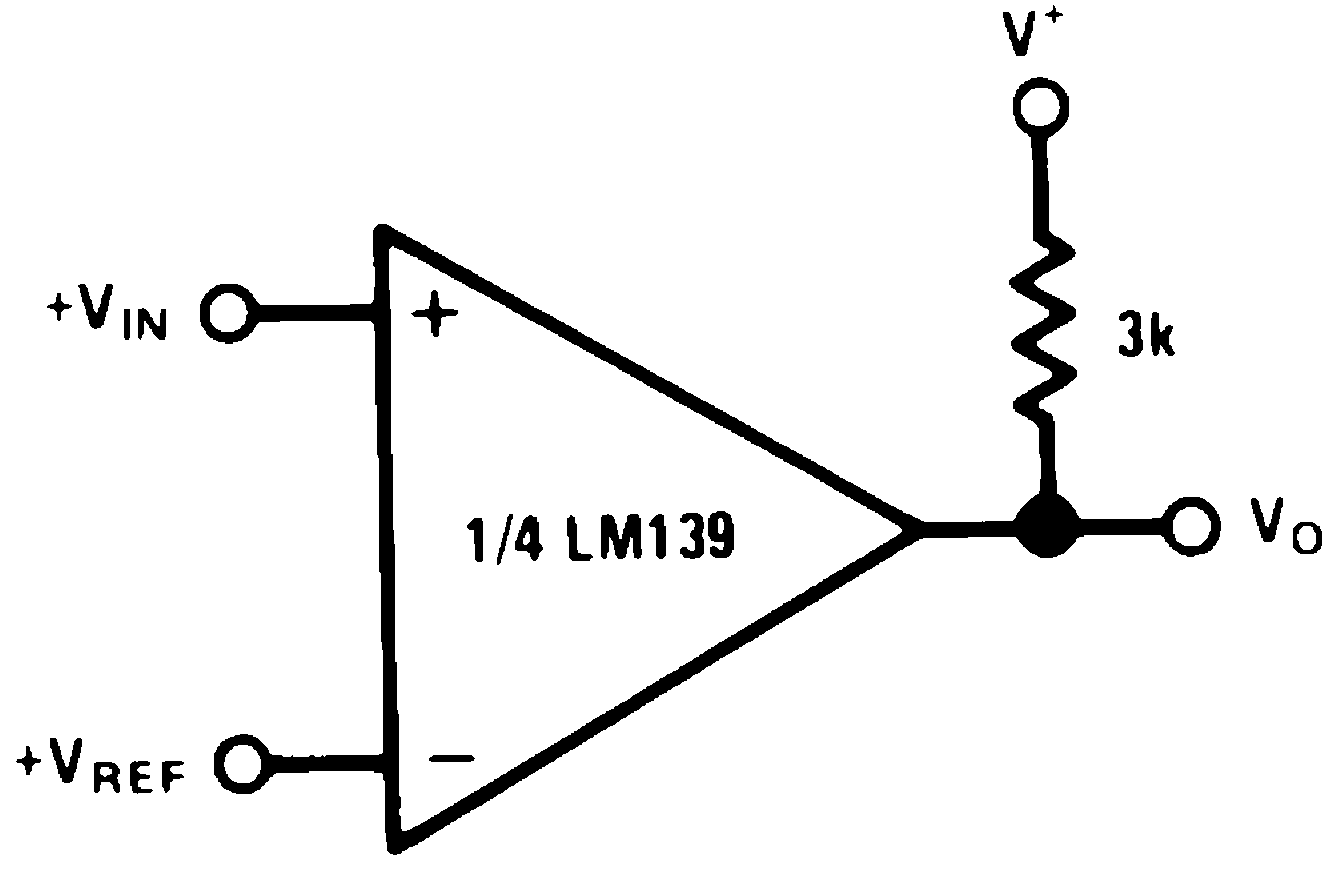Figure 22. Basic Comparator
(V+= 15 VDC)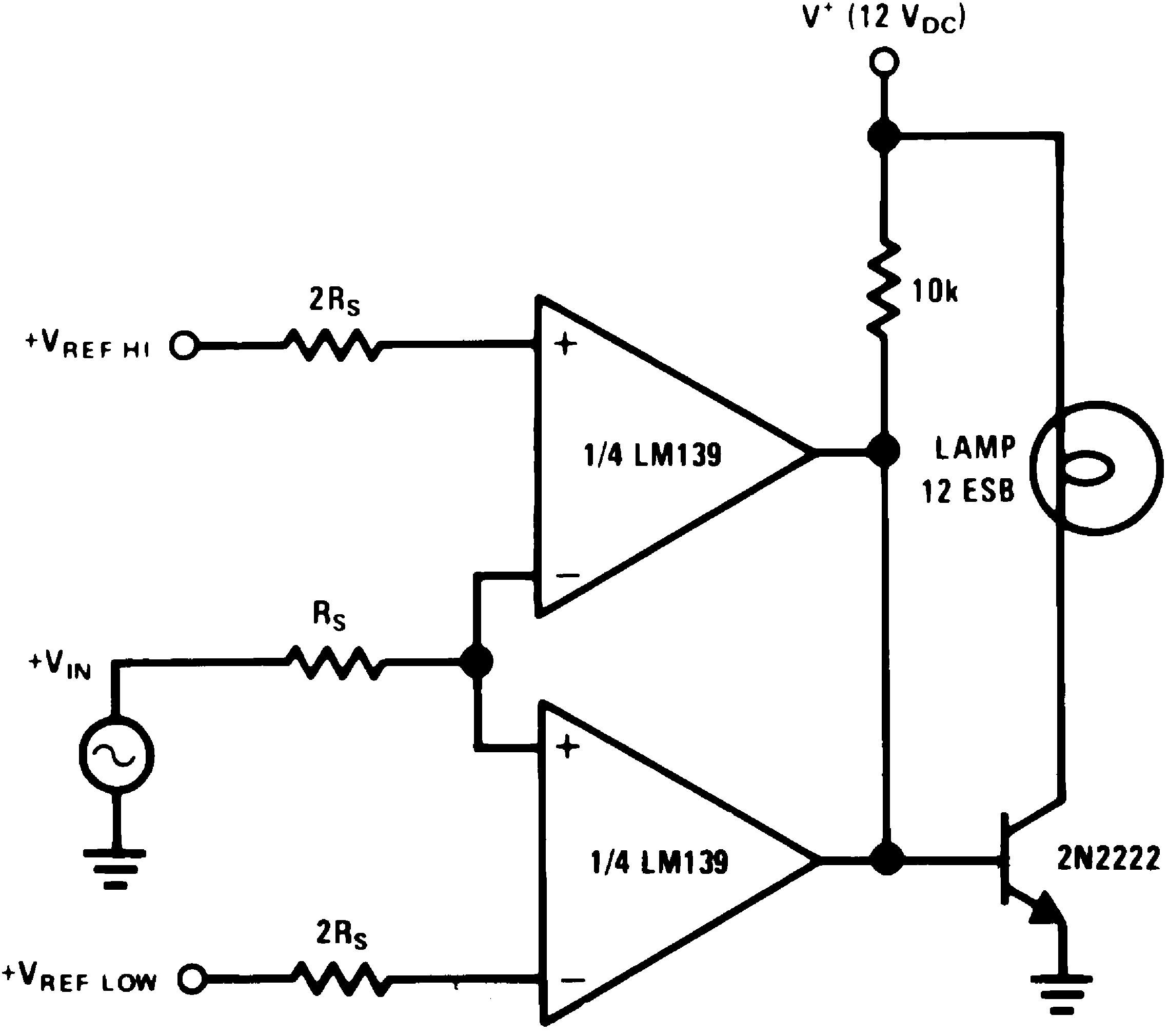Figure 23. Limit Comparator
(V+= 15 VDC)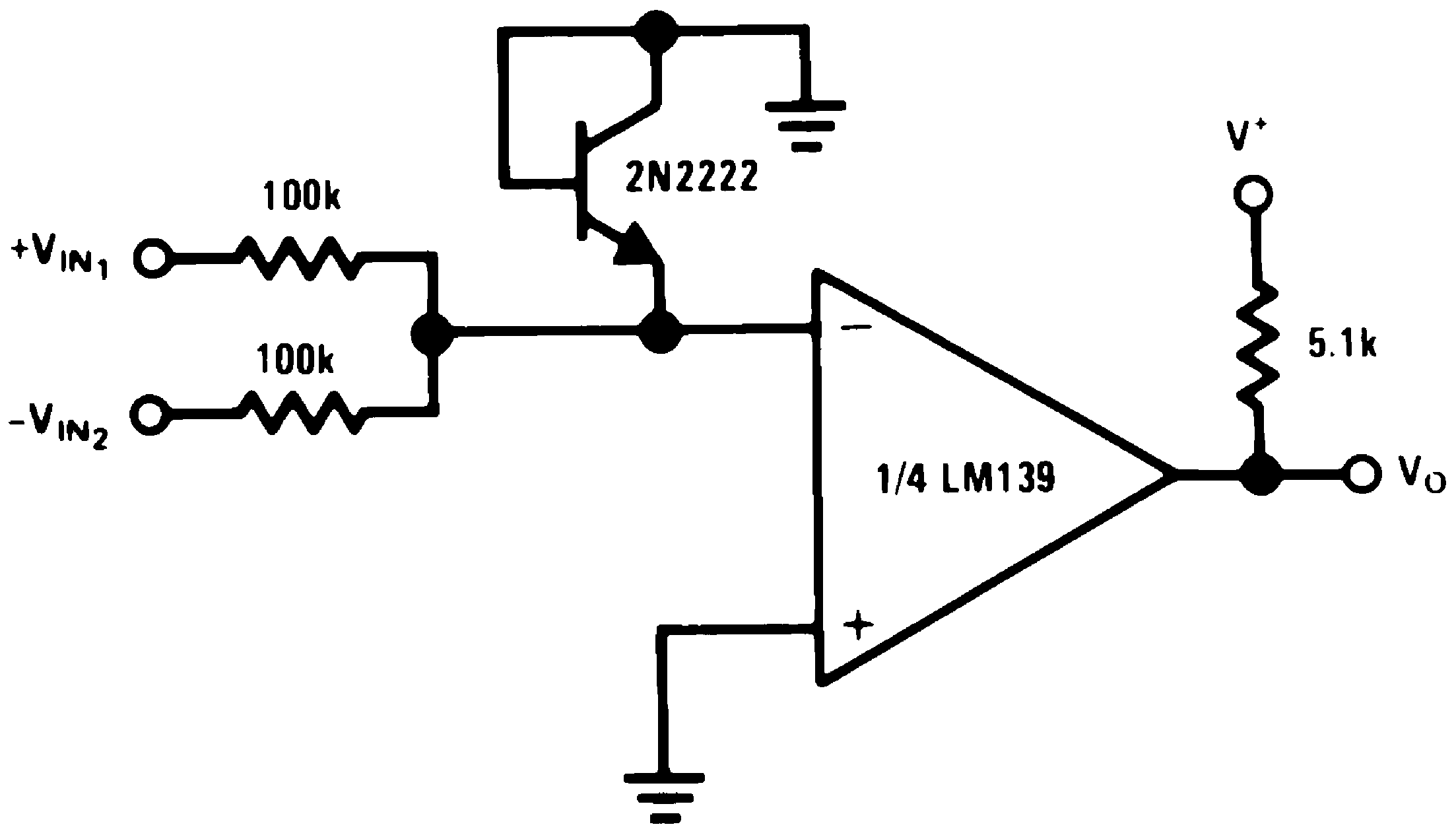Figure 24. Comparing Input Voltages of Opposite Polarity
(V+= 15 VDC)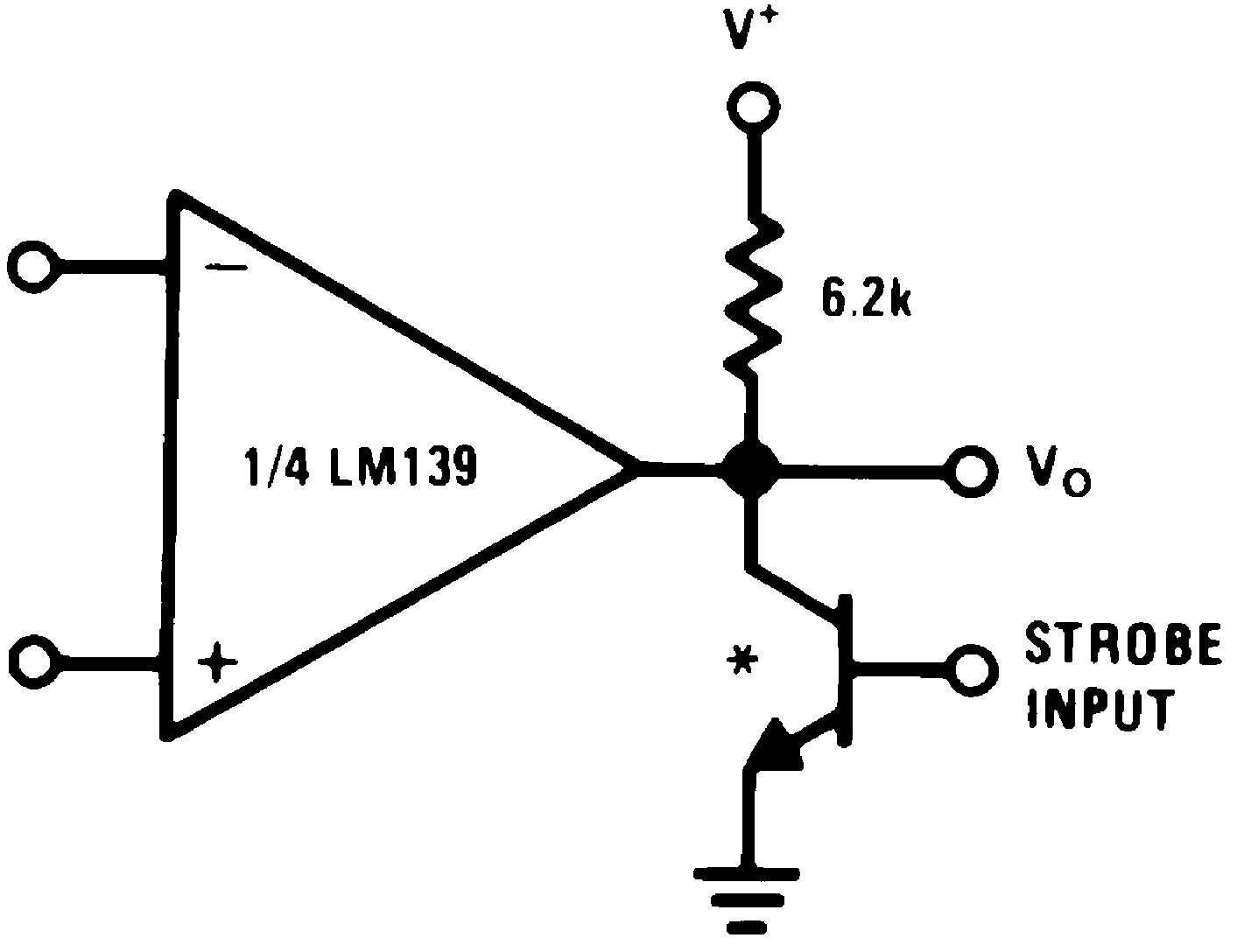* Or open-collector logic gate without pullup resistor
Figure 25. Output Strobing
(V+= 15 VDC)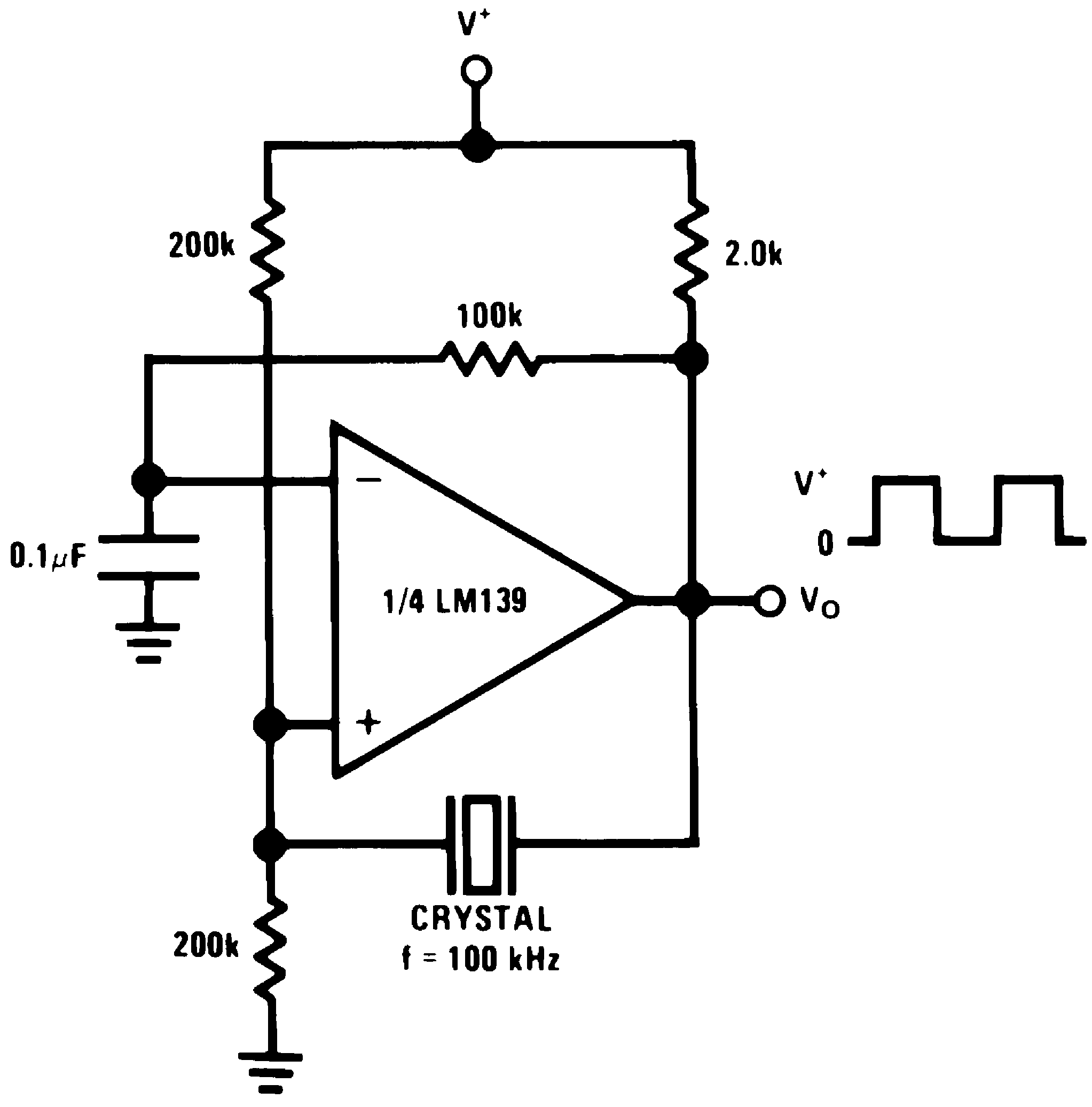Figure 26. Crystal Controlled Oscillator
(V+= 15 VDC)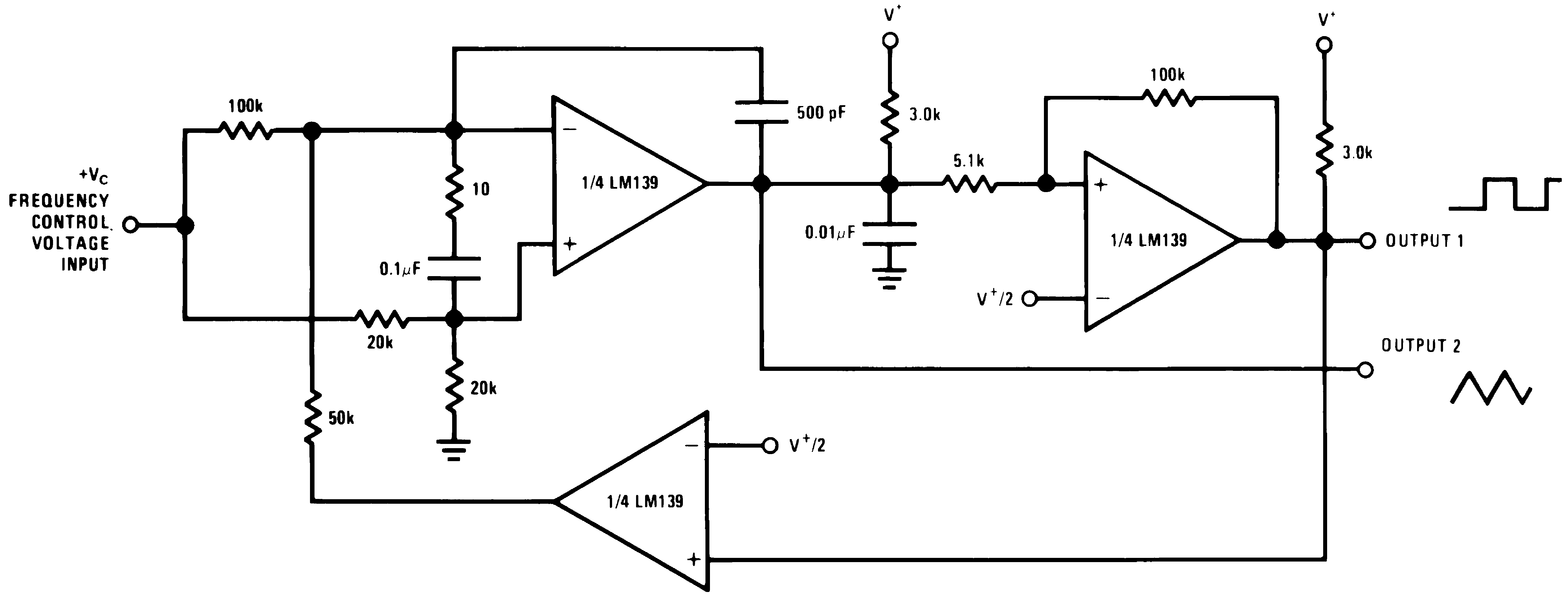250 mVDC ≤ VC ≤ +50 VDC 700 Hz ≤ fO ≤ 100 kHz
V+ = +30 VDC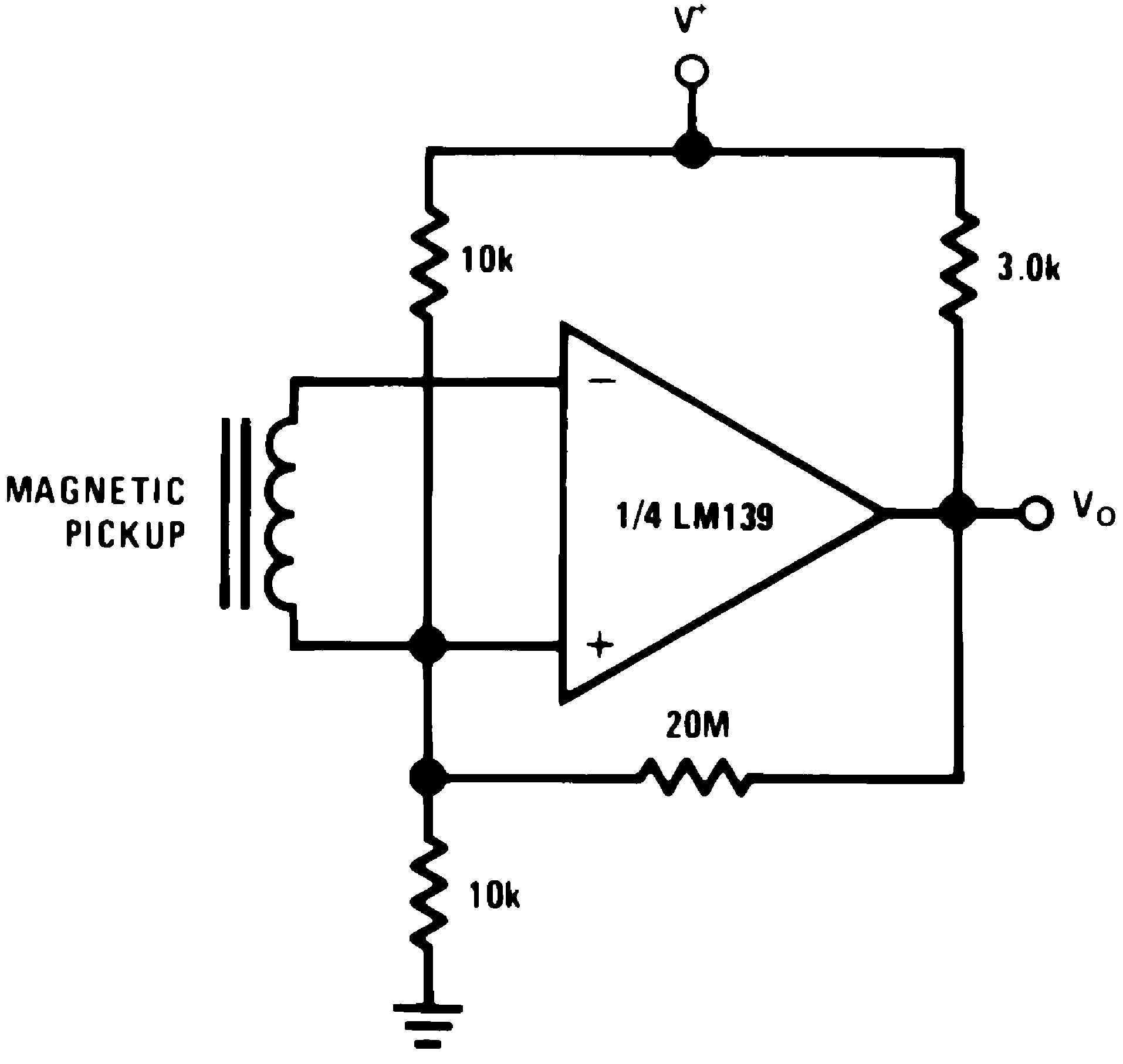Figure 28. Transducer Amplifier
(V+= 15 VDC)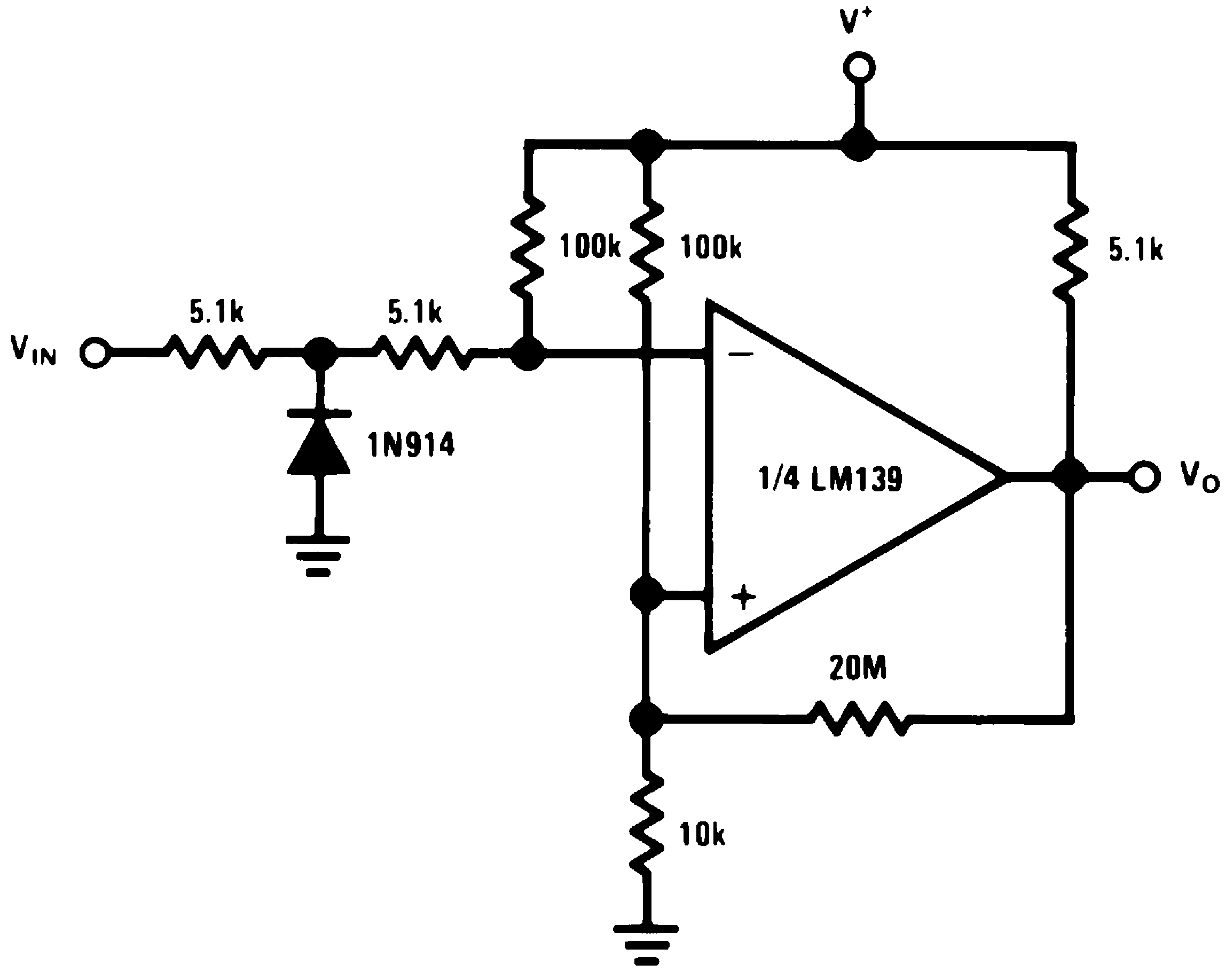Figure 29. Zero Crossing Detector (Single Power Supply)
(V+= 15 VDC)

#### 8.3.1 Split-Supply Applications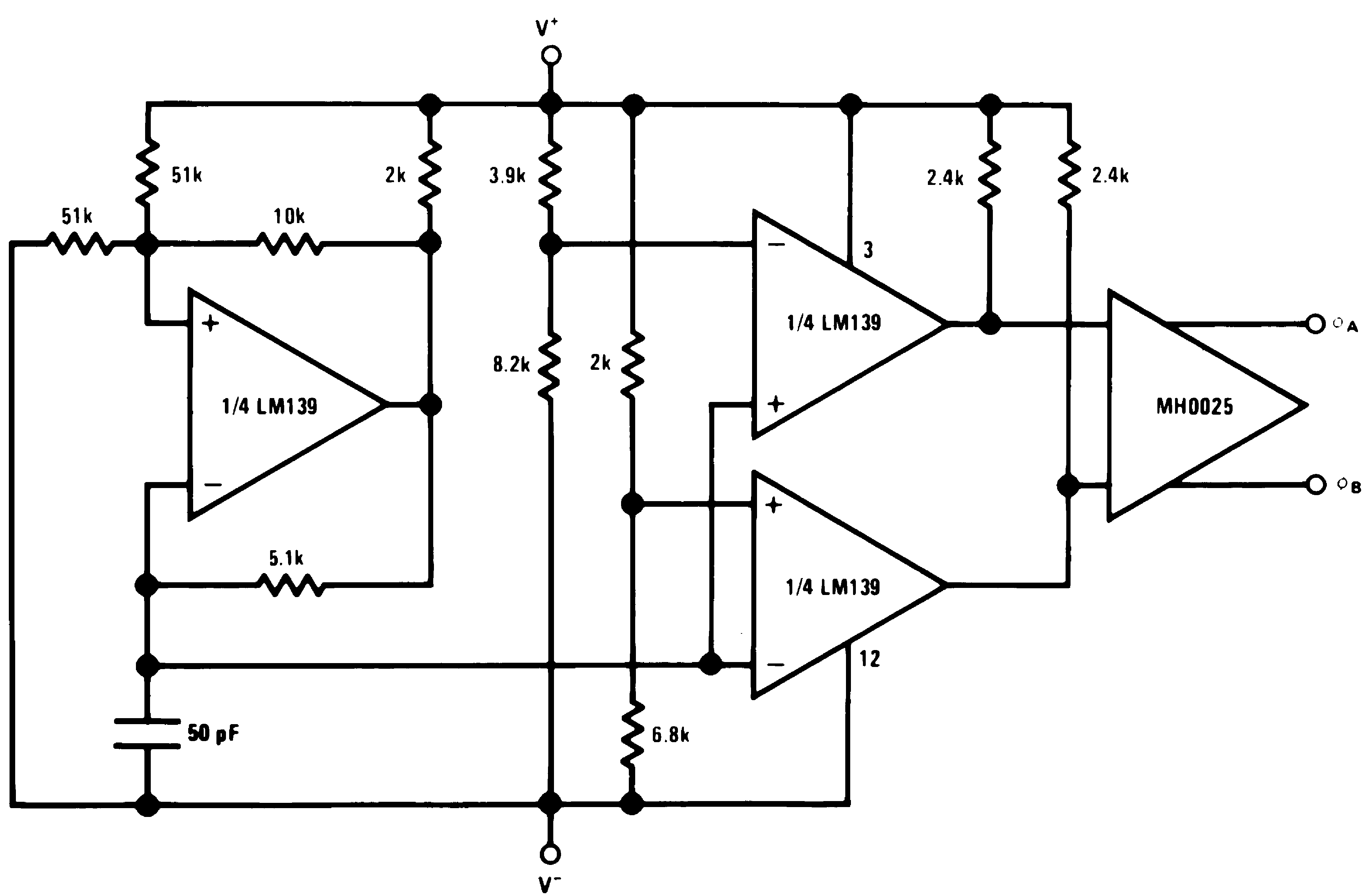Figure 30. MOS Clock Driver
(V+ = +15 VDC and V– = −15 VDC)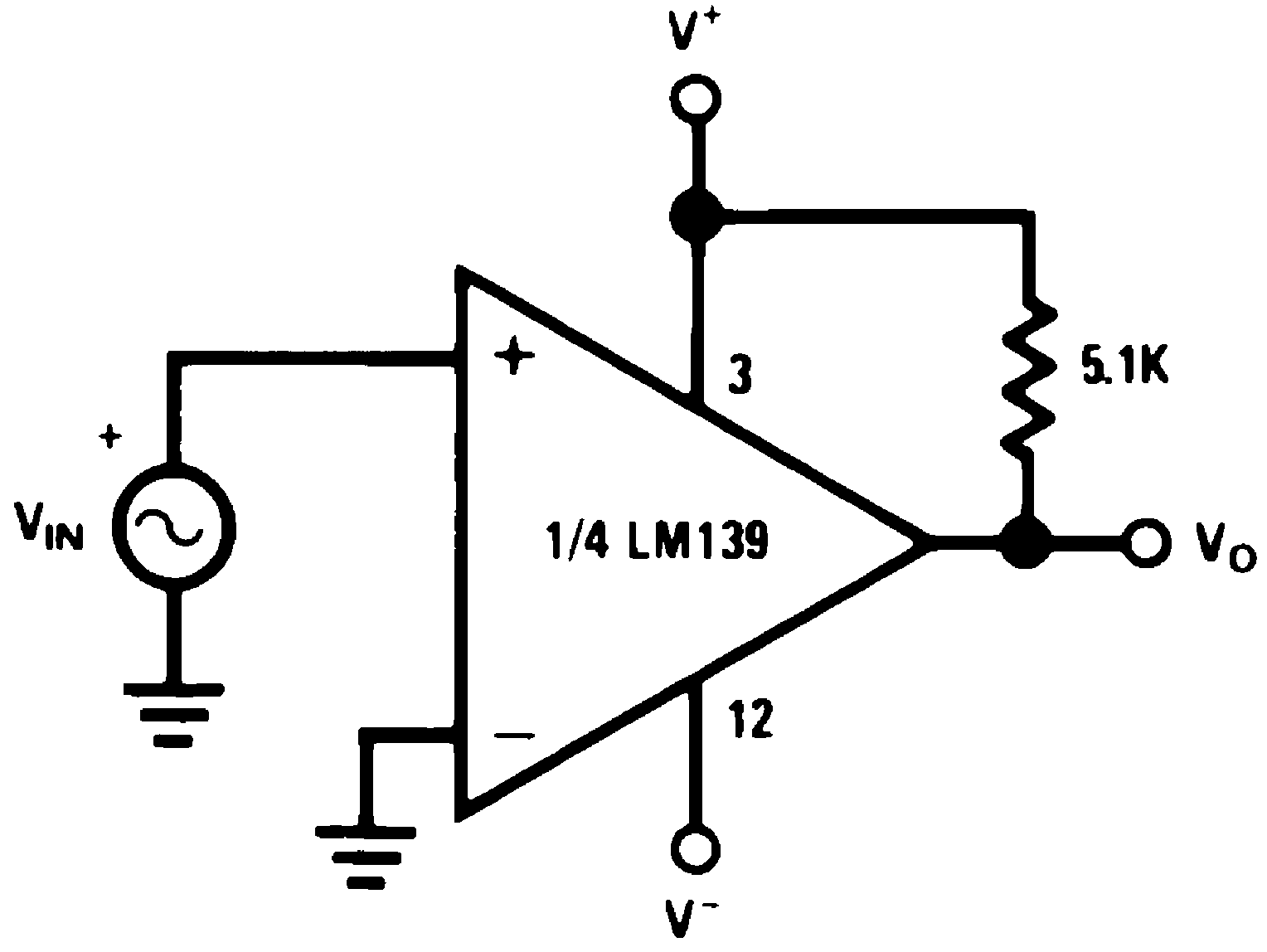Figure 31. Zero Crossing Detector
(V+ = +15 VDC and V– = −15 VDC)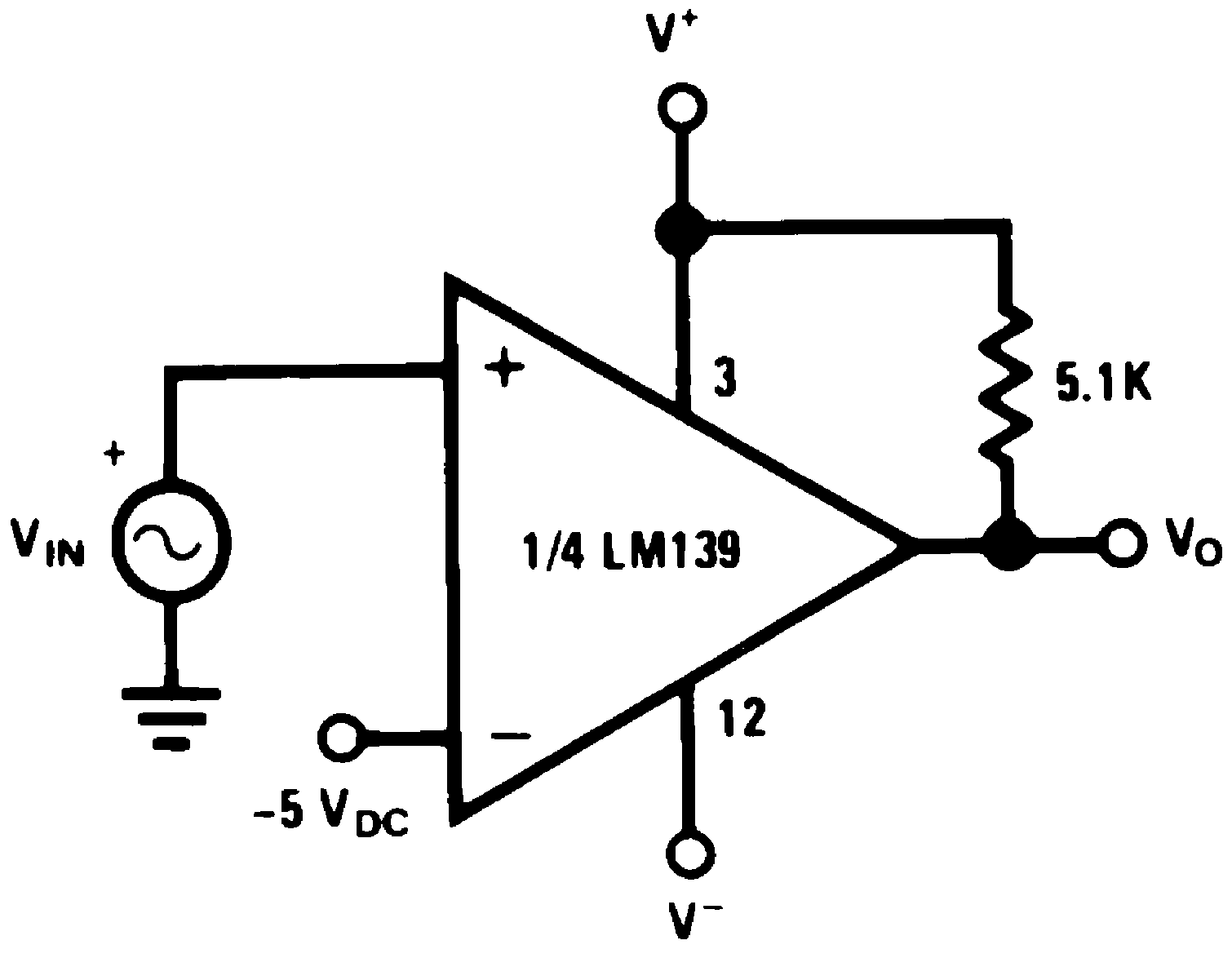Figure 32. Comparator With a Negative Reference
(V+ = +15 VDC and V– = −15 VDC)